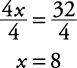## Ratios and Proportions

Ratios and proportions are not only used in arithmetic, but are also commonly used in algebra (and geometry).

#### Ratios

A ratio is a method of comparing two or more numbers or variables. Ratios are written as a:b or in working form, as a fraction.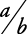or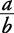is read “ a is to b

Notice that whatever comes after the “to” goes second or at the bottom of the fraction.

#### Proportions

Proportions are written as two ratios (fractions) equal to each other.

##### Example 1

Solve this problem for x.

p is to q as x is to y

First the proportion may be rewritten.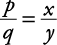Now simply multiply each side by y.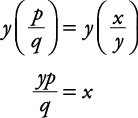##### Example 2

Solve this proportion for t.

s is to t as r is to q

Rewrite.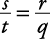Multiply each side of the equation by the common denominator, tq, and do early reducing.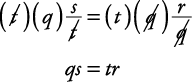Note that the result is the same as if you had “cross‐multiplied” through the equal sign. In future examples, this method is referred to as “cross‐multiply.”

Divide each side of the equation by r.#### Solving proportions for value

Follow the procedures given in Examples 10 and 11 to solve for the unknown.

##### Example 3

Solve for x.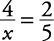Cross multiply.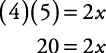Divide each side of the equation by 2.##### Example 4

Solve for x.Cross multiply.

5(2 x – 4) = 2(3 x + 6)

Use the distributive property.

10 x – 20 = 6 x + 12

Subtract 6 x from each side of the equation.Add 20 to each side of the equation.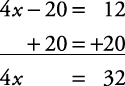Divide each side of the equation by 4.